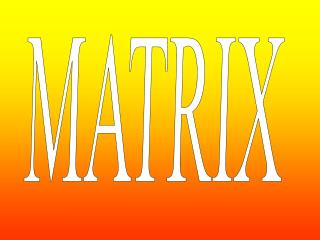DownloadDownload PresentationMATRIX

# MATRIX

Télécharger la présentation## MATRIX

- - - - - - - - - - - - - - - - - - - - - - - - - - - E N D - - - - - - - - - - - - - - - - - - - - - - - - - - -
##### Presentation Transcript

1. MATRIX

2. CONCEPT OF MATRIX • 1.Definition of matrix Equations Rectangular number table can be formed from the coefficients

3. This is a matrix The rectangular number table with m rows and n columns constructed by mn numbers in a certain order is called a mn Matrix (briefly matrix). The horizontals are called the rows of matrix and the verticals are called the columns of matrix Is called the element located row i and column j. The matrix is called real matrix if its elements are real numbers.From now on, we only discuss the real matrix.

4. Matrix is usually denoted with capital A、B、 C and so on. For instance Simply write: Column matrix Footmark Row matrix

5. The main diagonal line The matrix is called a square matrix if its row number is equal to its column number, i.e., m=n.

6. Several special matrixes

7. 6.Trapezoid matrix Let If holds for i>j (i<j), and the number of zero before (behind) the first (last) nonzero element is growing larger (less) as the rows increase, then we call A a upper (lower) trapezoid matrix. They are all called trapezoid matrixes.

8. ??? No! Are they Trapezoid matrixes? Please remember the characteristics of trapezoid matrix and follow its definition. Trapezoid matrix is the most frequently-used matrix.

9. Operation of matrix 一、linear operation 1.Equality: equality of two matrixes means that their number of rows and number of columns are respectively equal and their corresponding elements are the same. i.e., = corresponding elements are the same same type Type is the same

10. 2.addition、subtraction define Let and Obviously, A+B=B+A (A+B)+C=A+(B+C) A+O=O+A=A A-A=O Negative matrix: the negative matrix of is We write-A, i.e.,

11. 3.number multiple Is called the product of number and matrix， Briefly number multiple。write：kA

12. = In general，we have

13. what condition can enable A and B to multiply? = O Obviously It is the difference between Matrix and number.

14. Example2 But Please remember： 1.The multiplication of matrixes does not satisfy commutative law; 2. does not satisfy cancellation law； 3. Has nonzero zero factor. This is another difference between matrix and number.

15. Please particularly notice property 5. The conclusion does not hold if the orders of A and B are not equal.

16. Positive integer power of the square matrix. Transposition of matrix. Please remember!

17. = = That is

18. Symmetric and anti-symmetric matrix Any square matrix can be decomposed to the sum of a symmetric matrix and an anti-symmetric matrix. The determinant of an odd order anti-symmetric matrix equals to zero. 0

19. Example 1：seek the power of matrix